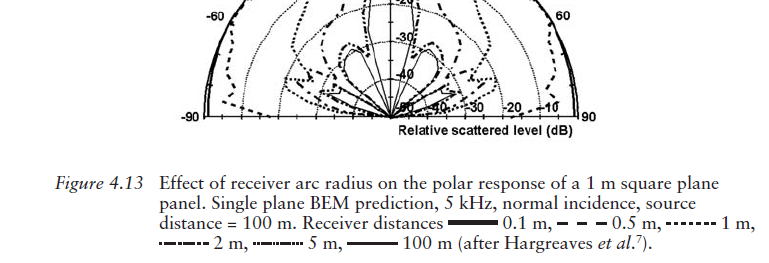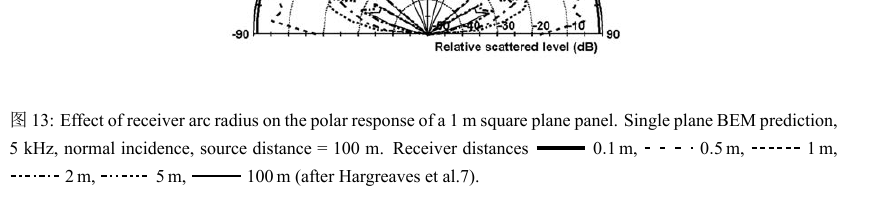•   OpenCV中，可以画圆、线、矩形、椭圆和多边形，但并不能画出虚线，现希望通过OpenCV已有的函数画出由点或线组成的虚线。 cv::circle() // 画一个简单圆 cv::clipLine() // 判断一条直线是否给定的矩形内 cv:...

一、背景
在OpenCV中，可以画圆、线、矩形、椭圆和多边形，但并不能画出虚线，现希望通过OpenCV已有的函数画出由点或线组成的虚线。
cv::circle() // 画一个简单圆
cv::clipLine() // 判断一条直线是否在给定的矩形内
cv::ellipse() // 画一个椭圆，可以倾斜，或者只有部分圆弧
cv::ellipse2Poly() // 计算一个近似椭圆的多边形
cv::fillConvexPoly() // 画一个填充的简单多边形
cv::fillPoly() // 画一个填充的任意多边形
cv::line() // 画一条简单直线
cv::rectangle() // 画一个简单矩形
cv::polyLines() // 画多重折线

二、实现
注意事项： 1、OpenCV虽然没有画点的函数，但可通过cv::circle()实现，只要半径够短，线够厚，一个实心圆可看成一个点。 2、创建空白图片时，其图片类型应选择CV_32FC3或CV_64FC3。存储像素的位数越多，画出的斜线越直。 3、两个点画出一条线，故使用g_first和g_last两个Point来存储所画的线。画线主要分为水平线、垂直线和倾斜线三种情况。倾斜线表示方法如下，设置每个点或线的间隔，已知

x

x

即可求出

y

y

，由此可确定每个点或每条线的坐标。

y

−

y

1

y

2

−

y

1

=

x

−

x

1

x

2

−

x

1

⇒

y

=

(

y

2

−

y

1

)

(

x

2

−

x

1

)

∗

(

x

−

x

1

)

+

y

1

\frac{y-y_1}{y_2-y_1} = \frac{x-x_1}{x_2-x_1} \Rightarrow y = \frac{(y2-y1)}{(x2-x1)}*(x-x1)+y1

操作方法： 1、运行程序，在空白图片上按下鼠标左键并移动，鼠标左键释放时即可画出一条虚线。鼠标右键点击可擦掉已画出的虚线。 2、程序包含2种模式，模式1画出由点组成的虚线，模式2画出由线组成的虚线。鼠标默认使用模式1，可通过键盘输入1或2选择不同模式。键盘输入除1或2的键会自动退出程序。
#include <opencv2/opencv.hpp>
#include <iostream>
using namespace std;
using namespace cv;

Point2f g_first, g_last; // 线的起点和终点

// 画由点组成的虚线
void draw_dotted_line1(Mat img, Point2f p1, Point2f p2, Scalar color, int thickness)
{
float n = 15; //虚点间隔
float w = p2.x - p1.x, h = p2.y - p1.y;
float l = sqrtf(w * w + h * h);
int m = l / n;
n = l / m; // 矫正虚点间隔，使虚点数为整数

circle(img, p1, 1, color, thickness); // 画起点
circle(img, p2, 1, color, thickness); // 画终点
// 画中间点
if (p1.y == p2.y) // 水平线：y = m
{
float x1 = min(p1.x, p2.x);
float x2 = max(p1.x, p2.x);
for (float x = x1 + n; x < x2; x = x + n)
circle(img, Point2f(x, p1.y), 1, color, thickness);
}
else if (p1.x == p2.x) // 垂直线, x = m
{
float y1 = min(p1.y, p2.y);
float y2 = max(p1.y, p2.y);
for (float y = y1 + n; y < y2; y = y + n)
circle(img, Point2f(p1.x, y), 1, color, thickness);
}
else // 倾斜线，与x轴、y轴都不垂直或平行
{
// 直线方程的两点式：(y-y1)/(y2-y1)=(x-x1)/(x2-x1) -> y = (y2-y1)*(x-x1)/(x2-x1)+y1
float m = n * abs(w) / l;
float k = h / w;
float x1 = min(p1.x, p2.x);
float x2 = max(p1.x, p2.x);
for (float x = x1 + m; x < x2; x = x + m)
circle(img, Point2f(x, k * (x - p1.x) + p1.y), 1, color, thickness);
}
}

// 画由线组成的虚线
void draw_dotted_line2(Mat img, Point2f p1, Point2f p2, Scalar color, int thickness)
{
float n = 15; //线长度
float w = p2.x - p1.x, h = p2.y - p1.y;
float l = sqrtf(w * w + h * h);
// 矫正线长度，使线个数为奇数
int m = l / n;
m = m % 2 ? m : m + 1;
n = l / m;

circle(img, p1, 1, color, thickness); // 画起点
circle(img, p2, 1, color, thickness); // 画终点
// 画中间点
if (p1.y == p2.y) //水平线：y = m
{
float x1 = min(p1.x, p2.x);
float x2 = max(p1.x, p2.x);
for (float x = x1, n1 = 2 * n; x < x2; x = x + n1)
line(img, Point2f(x, p1.y), Point2f(x + n, p1.y), color, thickness);
}
else if (p1.x == p2.x) //垂直线, x = m
{
float y1 = min(p1.y, p2.y);
float y2 = max(p1.y, p2.y);
for (float y = y1, n1 = 2 * n; y < y2; y = y + n1)
line(img, Point2f(p1.x, y), Point2f(p1.x, y + n), color, thickness);
}
else // 倾斜线，与x轴、y轴都不垂直或平行
{
// 直线方程的两点式：(y-y1)/(y2-y1)=(x-x1)/(x2-x1) -> y = (y2-y1)*(x-x1)/(x2-x1)+y1
float n1 = n * abs(w) / l;
float k = h / w;
float x1 = min(p1.x, p2.x);
float x2 = max(p1.x, p2.x);
for (float x = x1, n2 = 2 * n1; x < x2; x = x + n2)
{
Point p3 = Point2f(x, k * (x - p1.x) + p1.y);
Point p4 = Point2f(x + n1, k * (x + n1 - p1.x) + p1.y);
line(img, p3, p4, color, thickness);
}
}
}

// 矫正坐标
void correct(Point2f &p, const Point2f &p1, const Point2f &p2)
{
if (p.x < p1.x)
p.x = p1.x;
else if (p.x > p2.x)
p.x = p2.x;
if (p.y < p1.y)
p.y = p1.y;
else if (p.y > p2.y)
p.y = p2.y;
}

// 鼠标回调函数
void mouse_callback(int event, int x, int y, int flags, void *param)
{
static size_t i = 0; // 鼠标操作步骤的标识
switch (event)
{
case cv::EVENT_LBUTTONDOWN: // 鼠标左键点击
if (i == 0)
{
g_first = Point2f(x, y);        // 保存线的起点
g_last = Point2f(x + 1, y + 1); // 保存线的终点
i = 1;
}
break;
case cv::EVENT_MOUSEMOVE: // 鼠标移动
if (i == 1)
g_last = Point2f(x, y); // 刷新线的终点
break;
case cv::EVENT_LBUTTONUP: // 鼠标左键释放
if (i == 1)
i = 2; // 不再画线
break;
case cv::EVENT_RBUTTONDOWN: // 鼠标右键点击，清除内容
i = 0;
g_first = Point2f(0, 0);
g_last = Point2f(0, 0);
break;
default:
break;
}
}

int main()
{
const int w = 800, h = 600;
const string window_name = "image";
Mat image_original = cv::Mat(h, w, CV_32FC3, cv::Scalar(255, 255, 255)); // 选择CV_32FC3，画出的斜线更直
cv::imshow(window_name, image_original);
cv::setMouseCallback(window_name, mouse_callback); // 鼠标函数
char flag = '1';
while (true)
{
Mat img = image_original.clone(); // 克隆图片，方便在同一张图片上多次画线
correct(g_last, Point2f(0, 0), Point2f(img.cols, img.rows));
if (flag == '1')
draw_dotted_line1(img, g_first, g_last, Scalar(0, 255, 0), 2); // 模式1画由点组成的虚线
else
draw_dotted_line2(img, g_first, g_last, Scalar(0, 255, 0), 2); // 模式2画由线组成的虚线
imshow(window_name, img);

char c = waitKey(1); // 获取键盘输入的字符
if (c == '1' || c == '2')
flag = c;
else if (c > 0) // 若不按'1'或'2'，按其它字符键会自动退出。
break;
}
return 0;
}

运行结果：展开全文opencv
• 有些中文期刊 (如《统计研究》) 要求图片中的网格线为虚线，而不是 Stata 默认的浅灰色实线。我们可以使用yline()选项自行修改。 Stata 范例 代码 cd "D:\stata15\ado\personal\Jianshu\graph_虚线网格线" *-...
全文阅读：https://www.lianxh.cn/news/126f5d3d6fae5.html

作者：连玉君 (中山大学)E-Mail: arlionn@163.com

简介
有些中文期刊 (如《统计研究》) 要求图片中的网格线为虚线，而不是 Stata 默认的浅灰色实线。我们可以使用 yline() 选项自行修改。
Stata 范例
代码

*-数据预处理
. sysuse "auto.dta", clear
. gen t = _n + 1999
. keep in 1/10
. replace price = price/100

*-图形模板
. set scheme s1mono
. twoway connected price t
. graph export "Fig1_无网格线.png", replace

. twoway connected price t, ///
ylabel(, grid)
. graph export "Fig2_添加网格线_默认.png", replace

. twoway connected price t, ///
ylabel(, nogrid) ///
yline(40(20)100, lp(dash) lc(black*0.4))
. graph export "Fig3_添加网格线_虚线.png", replace

全文阅读：https://www.lianxh.cn/news/126f5d3d6fae5.html
展开全文stata 连享会
• 实例如下： $(function () {$('ul li').addClass(function (i) { return i % 6 == 5 ? "ab" : ""; }); // 隔6行 加空白。或者加虚线 \$('.imglist li').after(function (i) { ...图片隔三行加虚线，最后一
• 这是我国外社区里的一个提问，别人启发下解决了问题，转发到这里，也算是原创吧。想要这样的效果 根据提示使用tikz包，但是不兼容，发现问题处在，\caption里所有的命令都要加上\protect最终代码如下\begin{...
这是我在国外社区里的一个提问，在别人启发下解决了问题，转发到这里，也算是原创吧。
想要这样的效果根据提示使用tikz包，但是不兼容，发现问题处在，\caption里所有的命令都要加上\protect
最终代码如下
\begin{figure*}[!htb]
\begin{center}
\includegraphics[width=0.4\paperwidth]{figures/4_13.jpg}
\end{center}
\caption{ \label{fig:4_13}Effect of receiver arc ...eiver distances
\protect\tikz[baseline]{\protect\draw[line width=1mm] (0,.8ex)--++(1,0) ;}~\SI{.1}{\meter},
\protect\tikz[baseline]{\protect\draw[line width=0.5mm,loosely dashed] (0,.8ex)--++(1,0) ;}~\SI{.5}{\meter},
\protect\tikz[baseline]{\protect\draw[line width=0.5mm,densely dashed] (0,.8ex)--++(1,0);}~\SI{1}{\meter},
\protect\tikz[baseline]{\protect\draw[line width=0.5mm,dash dot] (0,.8ex)--++(1,0) ;}~\SI{2}{\meter},
\protect\tikz[baseline]{\protect\draw[line width=0.5mm,dash dot dot] (0,.8ex)--++(1,0) ;}~\SI{5}{\meter},
\protect\tikz[baseline]{\protect\draw[line width=0.5mm] (0,.8ex)--++(1,0) ;}~\SI{100}{\meter} (after Hargreaves et al.7). }
\end{figure*}
最终效果如下：基本与原图一致，还有别的图的问题还未解决，tikz文档有一千多页，抽空研究一下再发上来。
展开全文latex figure caption
• 如何在图片上绘制两点之间虚线？  ....... m_GraView->setScene(m_GraScene); m_GraView->resize(this->width(), this->height()); m_GraView->setStyleSheet("background: black;border:0px"); m_GraScene...c++ c语言 java 开发语言
• 当点击一个a标签更换验证码，点击后a标签有个阴影部分，非常影响美观于是搜集了一些整理方法，比较实用，今天正好遇到。故记录于此。知识要靠一点一点积累，感兴趣的你可以参考下
• 控制台查看 el-tree 渲染后的HTML结构，找到相应的节点添加伪元素，利用伪元素的border属性加上定位实现虚线效果。 渲染后HTML结构如下，可发现树的结构是由一个类名为 el-tree-node 的div包裹着两个分别代表节点...
需求需要实现一个带有指示线的树形组件，项目用的Vue，树形组件使用的是element的el-tree，所以想直接在element的组件上直接改样式实现。 先看最终实现的效果图：
思路 在控制台查看 el-tree 渲染后的HTML结构，找到相应的节点添加伪元素，利用伪元素的border属性加上定位实现虚线效果。
渲染后HTML结构如下，可发现树的结构是由一个类名为 el-tree-node 的div包裹着两个分别代表节点本身和子节点集的div 递归遍历生成的，类名为 el-tree-node 的div就是一个单位。所以我们只要在这个div上添加伪元素并修改样式就可以实现对应的虚线了。
遇到问题 按照上述的思路去实现，你会发现每一层的虚线无法根据缩进正确地定位，原因就是树形的每一层 el-tree-node 的宽度都是撑满整个树形大盒子的（如下图），我们的伪元素无法根据当前层的缩进数去定位。
解决办法 element的官网由提供一个属性 indent ，该属性可以控制相邻级节点间的水平缩进，单位为像素。我们可以将该属性设置为0，然后我们在css中为所有 el-tree-node 设置 padding-left ，就可以达到缩进的效果了！
由于每一层 el-tree-node 都被上一层的 el-tree-node 包裹着，上一层的padding会影响到下层的div位置，所以每一层的节点就不会撑满整个树形大盒子了，现在就可以直接根据 el-tree-node 定位伪元素了。
实现代码与注释
<el-tree
class="tree-line"
icon-class="el-icon-circle-plus-outline"
:indent="0"
:data="data"
></el-tree>
// 以下为scss，记得去掉scoped，或者使用/deep/
<style lang="scss">
.tree-line{
.el-tree-node {
position: relative;
}
.el-tree-node__children {
}

// 竖线
.el-tree-node::before {
content: "";
height: 100%;
width: 1px;
position: absolute;
left: -3px;
top: -26px;
border-width: 1px;
border-left: 1px dashed #52627C;
}
// 当前层最后一个节点的竖线高度固定
.el-tree-node:last-child::before {
height: 38px; // 可以自己调节到合适数值
}

// 横线
.el-tree-node::after {
content: "";
width: 24px;
height: 20px;
position: absolute;
left: -3px;
top: 12px;
border-width: 1px;
border-top: 1px dashed #52627C;
}

// 去掉最顶层的虚线，放最下面样式才不会被上面的覆盖了
& > .el-tree-node::after {
border-top: none;
}
& > .el-tree-node::before {
border-left: none;
}

// 展开关闭的icon
.el-tree-node__expand-icon{
font-size: 16px;
// 叶子节点（无子节点）
&.is-leaf{
color: transparent;
// display: none; // 也可以去掉
}
}
}
</style>
———————————————— 版权声明：本文为CSDN博主「吕大瓜」的原创文章，遵循CC 4.0 BY-SA版权协议，转载请附上原文出处链接及本声明。 原文链接：https://blog.csdn.net/weixin_42490398/article/details/107942461`
展开全文• ## 微信小程序加虚线

万次阅读 2018-12-05 18:19:16
.cartLeft{ width: 50%; height: 100rpx; border-right: 1rpx dashed #a7a8a8; }
• 【R语言系列02】R作图中的标注虚线，文本，阴影误差带 【以往专题】 【R语言系列01】拼贴操作 paste 与 paste0 本期将从几个模拟实例当中提供一些常用作图技巧的分享。 需要的包： reshape2进行长宽表转换 ggplot2 ...ggplot2 可视化 r语言
• 一篇介绍了图片渐变蒙版处理，还有一些其他的小技巧，一起这里介绍一下。可以直接看目录标题，几个方法相互独立。 文章目录PIL处理图片虚线前言一、pandas是什么？二、使用步骤1.引入库2.读入数据总结 画虚线 ...python 图像处理
• ## Unity 画虚线

千次阅读 2017-11-18 01:38:40
• latex 表格中虚线When documents are digitalized via scanning or via photo, the image quality can suffer from wrong settings or bad conditions. In the case of table recognition, this can lead to a ...
• 设计和制作网页过程中，常常需要加入虚线分割线，像腾讯、搜狐、新浪首页都有灰色虚线的应用。但是虚线的实现，无论设计中还是后面的制作中都不太容易。 （一）设计中实现虚线 总结以下photoshop画虚线的几种...html5 html css css3
• drawable建xml文件，里面设置shape来设置文本框的特殊效果 &amp;lt;?xml version=&quot;1.0&quot; encoding=&quot;utf-8&quot;?&amp;gt; &amp;lt;shape xmlns:android=&quot;...
• 有图又真相，先图再说。点击效果：设置虚线：[html] view plaincopyxml version="1.0" encoding="utf-8"?> shape xmlns:android="http://schemas.android.com/apk/res/android" android:shape="line" > ...Android
• 一、下划虚线以背景形式默认状态下（下划虚线往往会自上而下形成） 代码：background:url(../images/hot_bottom_bg.gif) no-repeat; 例图： 二、下划虚线以背景形式设置状态下（下划虚线就会自下而上形成） ...
• 1.效果图   2.View添加虚线  （1）实现view子类 ，进行绘制 package labelnet.cn.ledou.ui; import android.content.Context; import android.graphics.Canimageview
• 总结了一下几种画虚线的方式。 ①使用LineRenderer ②代码生成网格画虚线 ③使用片元着色器画虚线 ④使用几何着色器画虚线 ⑤使用UILineRenderer ⑥使用Vectrosity插件unity3d shader 几何着色器
• 易语言图象阴影虚线的填充方式源码,图象阴影虚线的填充方式,取设备场景_,释放设备场景_,选入设备场景_,创建阴影刷子_,设置背景颜色_,画矩形_,设置背景模式_,删除对象_
• 用某些APP时，拍身份证照片会出现虚线框内，头部区域，国徽区域的引导框,请问下这些功能是怎么实现的呢？ 先谢过大家啦！android html5 前端
• ## css 虚线步宽的写法

千次阅读 2019-10-29 15:37:47
• 图如上图。 fn = '1.jpg'; p = imread(fn); imshow(p); [m,n,p] = size(p); hold on M = 10; % 水平分量 N = 10; % 垂直分量 lw = 0.1; % 划线宽度 mx= ones(1,M+1); ...for k = 1...
• 微信图文排版指南-如何在图片上添加文字？ 编辑排版公众号文章的时候，排版经常会选择图片和文字相结合，那如何在图片上添加文字呢？今天给大家分享两种办法~~ 一、给文字添加背景 1、编辑区直接输入文字，文字...
• matlab画图各种虚线代码法国制造 法国制造的整洁项目清单 内容 JavaScript :glowing_star: 名称 描述 :globe_showing_Europe-Africa: 6408 / 专门用于图形绘制JavaScript库 5964 / Bootstrap 3的AngularJS 1.2+本机...
• Indent Guides ... vs2013及以上直接扩展中搜索。 VS2010 - 12 http://indentguide.codeplex.com/releases/view/110312 下载对应版本的...
• 布局 &lt;R.color.dash_line.DashLineView android:id="@+id/dashLineView" android:layout_width="334dp"... android:layout_height="... android:layout_marginLe...
• //图片路径  BufferedImage image = ImageIO.read(new File("d:\\test.jpg"));  Graphics g = image.getGraphics();  g.setColor(Color.RED);//画笔颜色  g.drawRect(100, 100, 100, 100);//矩形框...java...